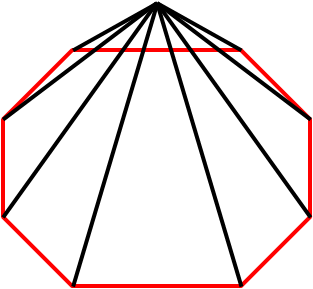#### You may also like### Building Tetrahedra

Can you make a tetrahedron whose faces all have the same perimeter?A 1 metre cube has one face on the ground and one face against a wall. A 4 metre ladder leans against the wall and just touches the cube. How high is the top of the ladder above the ground?Four rods are hinged at their ends to form a convex quadrilateral. Investigate the different shapes that the quadrilateral can take. Be patient this problem may be slow to load.

# Pyramidal N-gon

##### Age 14 to 16 ShortChallenge LevelThe base of the pyramid has $n$ edges, so also has $n$ vertices around the base. This then means that there are $n$ edges around the base (in red) of the pyramid and $n$ that meet at the apex (in black).

Therefore there are $2n$ edges in total.

There are also $n$ faces that meet at the apex of the pyramid, and one more for the base, so a total of $n+1$ faces.

Therefore the difference is $2n - (n+1) = n-1$.
This problem is taken from the UKMT Mathematical Challenges.
You can find more short problems, arranged by curriculum topic, in our short problems collection.